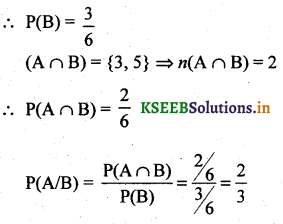# 2nd PUC Basic Maths Question Bank Chapter 3 Probability

Students can Download Basic Maths Question Bank Chapter 3 Probability Questions and Answers, Notes Pdf, 2nd PUC Maths Question Bank with Answers helps you to revise the complete Karnataka State Board Syllabus and to clear all their doubts, score well in final exams.

## Karnataka 2nd PUC Basic Maths Question Bank Chapter 3 Probability

### 2nd PUC Basic Maths Probability One or Two Marks Questions and Answers

Question 1.
State Multiplication theorem?
If A and B are events then P(A∩B) = P(A). P(B/A).

Question 2.
What do you mean by conditional probability?
The probability that an event B occurs once A has occurred is called the conditional probability of B given A.

Question 3.
Two coins are tossed. What is the probability that both are tails.
S = {HH, HT, TH, TT}
n(S) = 4  A={TT}
n(A) =1
∴ p(both tails) $$=\frac{n(\mathrm{A})}{n(\mathrm{S})}=\frac{1}{4}$$Question 4.
Three coins are tossed. What is the probability that they all fall heads?
S = {HHH, HHT, HTH, THH, HTT, THT, TTH, TTT}
n(s) = 8
A = {HHH}
n(A) = 1
$${ P }({ A })=\frac { n({ A }) }{ n({ S }) } =\frac { 1 }{ 8 }$$

Question 5.
A die is thrown once. what is the probability of getting the number 6
S = {1,2,3,4,5,6}
n(S) = 6
A = {6}
n(A) = 1
$${ P }({ A })=\frac { n({ A }) }{ n({ S }) } =\frac { 1 }{ 6 }$$

Question 6.
Two dice are thrown together. What is the Probability of getting a sum of 6?
A = {(3,3) (1,5) (5,1) (2,4) (4, 2)}
n(A) = 5
$$P(A)=\frac{n(A)}{n(S)}=\frac{5}{36}$$Question 7.
Two dice are thrown together. What is the Probability of getting a sum of 10.
n(s) = 36
A= {(5,5)(4,6)(6,4)}
n(A) = 3
$$P(A)=\frac{n(S)}{n(A)}=\frac{3}{36}=\frac{1}{12}$$

Question 8.
Find the Probability of getting a tail in a throw of a coin.
S = {H,T}
n(S) = 2
A = {T}
n(A) = 1
∴ p(A) = 1/2

Question 9.
If there are 2 children in a family. Find the Probability that there is atleast one girl child.
S = (BB, BQ GB, GG}
n(S) = 4
A={BB ,GB, GG}
n(A) = 3
$$P(A)=\frac{n(A)}{n(S)}=\frac{3}{4}$$

Question 10.
Two dice are rolled simultaneously. Find the probability of getting a doublet of even numbers.
Let w(S) = 6 x 6 = 36
A be the event of getting a doublet of even numbers
A = {(2,2) (4,4) (6, 6)}
n(A) = 3
$$P(A)=\frac{3}{36}=\frac{1}{12}$$Question 11.
T (P(A’) + P(A) = 1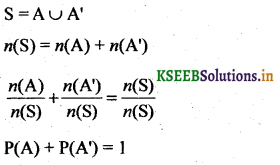Question 12.
A bag contains 8 red balls and 5 White balls. In a single draw of 3 balls. What is the Probability of drawing 3 white balls.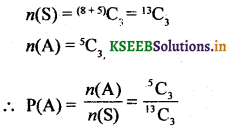Question 13.
If P(A) = 0.5, P(B) = 0.6 and P(A∪B) = 0.8 find P(A/B).
P(A∩B) = P(A) + P(B) – P(A∩B)
= 05 + 0.6 – 0.8
= 1.1- 0.8 P(A∩B)
$$P(A / B)=\frac{P(A \cap B)}{P(B)}=\frac{0.3}{0.6}=\frac{1}{2}=0.5$$Question 14.
Suppose a student is selected at random from 80 students, where 30 are taking Mathematics, 20 are taking Chemistry and 10 are taking Maths and Chemistry. Find the Probability of the student taking Mathematics or Chemistry.
Let A is a event that student takes Maths
B is an event that a student takes ChemistryQuestion 15.
If P(A) =$$\frac{1}{2}$$ ,P(B) =$$\frac{3}{5}$$ and P(A∩B)Question 16.
A bag contains 12 balls out of which 4 are red and 8 are green. 3 balls are drawn at random. What is the probability of getting all red balls?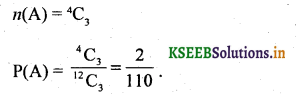Question 17.
From a set of 17 balls marked 1 to 17 one ball is drawn at random. What is the probability that it is multiple of 3 or 7
n (S)= 17
A = Multiple of 3 = {3, 6, 9, 12, 15}
n(A) = 5 and P(A) = $$\frac{5}{17}$$
B = Multiple of 7 = {7, 14}
n(B) = 2 and P(B) = $$\frac{2}{17}$$
A & B are mutually exclusive
∴ p(A∪B) = P(A) +p(B) = $$\frac{5}{17}+\frac{2}{17}=\frac{7}{17}$$Question 18.
P(A) = $$\frac{1}{4}$$, P(A∪B) = $$\frac{1}{2}$$ ,P(B) = $$\frac{2}{5}$$ . find P(A/B)Question 19.
Two cards are drawn from a pack of 52 cards. What is the Probability of drawing one King and one Jack.
n(S) = 52C2
There are 4 Kings and 4 Jacks out of which we have to choose 1 King and 1 Jack which can be chosen in 4C1 x 4C1 ways.
$$P(A)=\frac{^{4} C_{1} \times^{4} C_{1}}{^{52} C_{2}}=0.012$$Question 20.
Two cards are drawn from a pack of 52 well shuffled cards. Find the Probability that one is a heart and other is a spade.
We have to choose 2 cards out of 52 cards in 52C2 ways
n(S) = 52C2
We have one heart and one spade out of 13 heart and 13 spades in 13C1 & 13C1 ways
$$P(A)=\frac{^{13} C_{1} \times^{13} C_{1}}{^{52} C_{2}}=\frac{13}{102}=0.1272$$

Question 21.
If P(A) = 0.7, P(B) = 0.4, P(A∩B) = 0.3 find the value of P(A/B) and P(A’∩B’).
P(A∪B) = P(A) + P(B) – P(A∩B) = 0.7 + 0.4 – 0.3 = 0.8
P(A’∩B’) = P(A∪B)’ = 1 – P(A∪B)
= 1 – 0.8 = 0.2
∴ P(A’∩B’) = 0.2.

Question 22.
If A & B are 2 events such that P(A) = $$\frac{2}{3}$$, P(B) = $$\frac{2}{3}$$ and P(A∩B) = $$\frac{2}{3}$$. Find the Probability of the events A’ n B’ and A’∪B’Question 23.
The problem is given to three students Amar, Akbar and Anthony whose chances of solving it $$\frac{1}{2}, \frac{1}{3}, \frac{1}{4}$$ are respectively. If they try to solve a problem independently find the probability that the problem is not solved by anyone of them.Question 24.
In a group of 500 pens, 50 are found to be defective A Pen is selected at random from the batch. Find the probability that it is non defective.
As there are 50 defective pens the number of non defective pens is 450
We can choose one non defective pen in 450C1 ways.
$$P(A)=\frac{450}{500}=\frac{9}{10}$$Question 25.
A bag contains 7 red, 5 blue, 4 white and 4 black balls. A ball is drawn at random. Find the probability that the ball drawn is blue or black.
There are total 20 balls out of which one ball is chosen
Let A = Event that the ball is blue
∴ P(A) = $$\frac{5}{20}$$
Let B = Event that the ball is black
P(B) =  $$\frac{4}{20}$$
P(A or B) = P(A∪B) = P(A) + P(B)
$$=\frac{5}{20}+\frac{4}{20}=\frac{9}{20}$$

### 2nd PUC Basic Maths Probability Three or Four Marks Questions and Answers

Question 26.
One Ticket is drawn at random from a bag containing 30 tickets numbered from 1 to 30. Find the probability that it is a multiple of 3 or 5.
n(s) = 30
Let A = Multiple of 3 = {3, 6, 9, 12, 15, 18, 21, 24, 27, 30}
n(A) = 10
B = Multiple of 5 = {5, 10, 15, 20,25, 30}
n(B) = 6
A ∩ B = {15, 30}
n(A ∩ B) = 2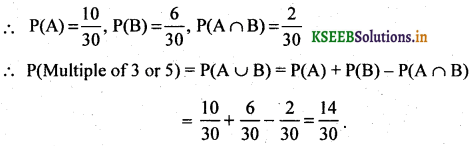Question 27.
Three students x ,y and z write the examination. Their chances of passing are $$\frac{1}{3}, \frac{1}{2} \text { and } \frac{1}{4}$$ respectively. Find the probability that at least one of them passess the examination.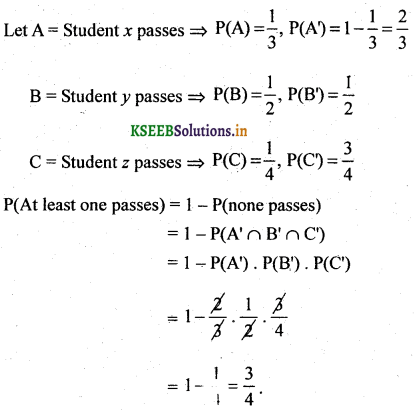Question 28.
A bag contains 4 white, 5 red and 6 black balls 2 balls are drawn at random from the bag. Find the Probability that one ball is black and other is white.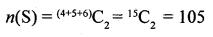we have the choose 1 black and 1 white ball which can be done in 4C1 x 6C1 x 5C0 ways = 4 x 6 x 1 = 24 ways =Question 29.
A box contains 8 tickets numbered 1,2,3,4,5,7,9,10. A ticket is drawn at random from the box and kept a side then the second ticket is drawn. Find the Probability that both the tickets drawn show odd numbers.
Let A be the event that the I ticket show an odd number and B be the event that the II ticket show an odd number
Both the tickets must be odd i.e. P(A∩B) = P(A).P(B/A)
there are 8 tickets out of which 5 are odd P(A) =$$\frac{5}{8}$$
Since event A taken place there are 7 tickets remaining out of which 4 are odd.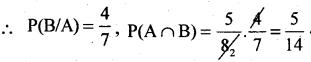Question 30.
Two dice are rolled. Find the Probability that the sum of the scores is a Prime number or a perfect square.
n(S) = 6 x 6 = 36
Let A be the event that the sum is a prime number
Let B be that the sum is a perfect square
A= {(1,1) (1,2), (1,4) (1,6) (2,1) (2,3) (2,5) (3,2) (3,4) (4, 1) (4,3) (5,2) (5,6) (6,1) (6,5)}
n(A)=15
∴ $${ P }({ A })=\frac { 15 }{ 36 }$$
B = {(1, 3) (3, 1) (3, 6) (6, 3) (4, 5) (5, 4)}
n(B) = 6
∴ $${ P }({ B })=\frac { 6 }{ 36 }$$
(A∩B) = 0    ∴ P(A∩B) = 0
P(A or B) = (A∪B) = P(A) + P(B) – P(A∩B)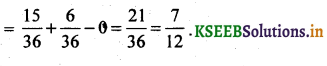Question 31.
Three coins are tossed simultaneously. State the sample space and mention the events A & B where (i) A is said to have occurred when an out come is 2 heads and 1 tail
B is said to have occurred when an outcome of the experiment is 2 or more heads. Find the Probability of A∪B.
S = {HHH, HHT, HTH, THH, HTT, TTH, THT, TTT}
w(S) = 8

(i) A = 2 Heads and 1 tail = {HHT, THH}
n(A) = 2
∴ $$P(A)=\frac{15}{36}$$

(ii) B = 2 or more heads = {HHH, HTH, HHT, THH}
n(B) = 4
∴ $$\frac{4}{8}=\frac{1}{2}$$
(A∩B) = {HHT, THH} n(A∩B) = 2
P(A∩B) = $$\frac{2}{8}=\frac{1}{4}$$
P(A∪B) = P(A) + P(B) – P(A∩B)  = $$\frac{1}{4}+\frac{1}{2}-\frac{1}{4}=\frac{1}{2}$$Question 32.
From well shuffled pack of 52 playing cards. 2 cards are drawn at random. Find the Probability that both the cards are spade cards or face cards.
n(S) = 52C2
Let A = be the event that both the cards are spade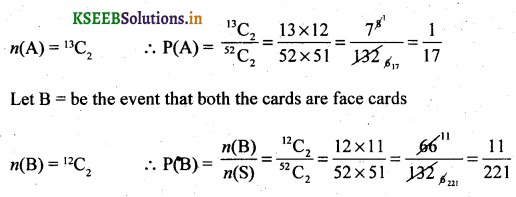Question 33.
Three cards are drawn at random from a well-shuffled pack of 52 playing cards. Find the Probability of getting a King, a Queen, and a Jack.
P(Event A) = P(getting a King) = $$\frac{^{4} C_{3}}{^{52} C_{3}}$$
P(Event B) = P(getting a Queen) = $$\frac{^{4} C_{3}}{^{52} C_{3}}$$
P(Event C) = P(getting a Jack) = $$\frac{^{4} C_{3}}{^{52} C_{3}}$$
Since A, B & C are independent P(A∩B∩C) = P(A).P(B). P(C)
$$\frac{^{4} C_{3}}{^52} \times \frac{^{4} C_{3}}{^{52} C_{3}} \times \frac{^{4} C_{3}}{^{52} C_{3}}$$

Question 34.
In a college, 35% of the student’s failed in Accountancy, 25% of the students failed in Commerce and 20% of the students failed in both. A student is selected at random. Find the Probability that (i) He failed in Accountancy, if he had failed in commerce (ii) He failed in commerce, if he had failed in Accountancy (iii) He failed in either of the two subjects.
A : Student fails in Accountancy
B : Student fails in Commerce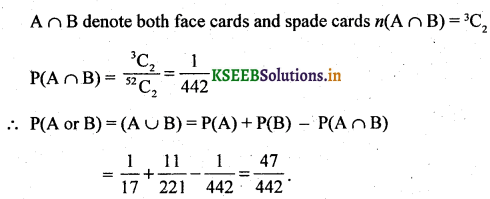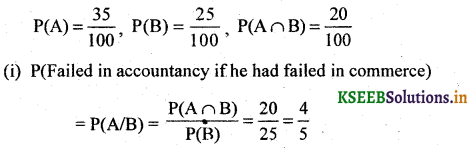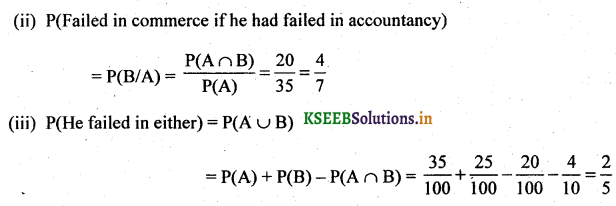Question 35.
A couple has 2 children. Find the Probability that both are boys. If it is known that
(i) one of the children is a boy
(ii) older child is a boy.
(i) S = {BQ GB, GG, BB}
Let A: Both the children are boys = {BB}
B : One of the child is a boy = {BQ GB, BB}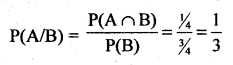(ii) A = Both the children are boys = {BB}, n(A) = 1
B = older child is a boy = {BG, BB}, n(B) = 2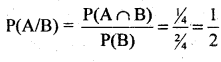Question 36.
Seven persons are to be seated in a row. Find the Probability that 2 particular persons sit next to each other.
Let A = Event that 2 persons are always seated together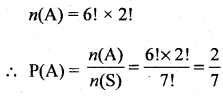Question 37.
Three fair coins are tossed simultaneously. Find the Probability of (i) getting one head (ii) getting almost one head (iii) getting atleast two head (iv) getting 3 heads when it is known that first two are heads.
S = {HHH, HHT, HTH, THH, HTT, THT, TTH, TTT}
n(s) = 8

(i) Let A = Event of getting one head
A = {HTT, THT, TTH} ⇒ n(A) = 3
∴ $$P(A)=\frac{3}{8}$$

(ii) Let B = Event of getting atmost one head
B = {HTT, THT, TTH, TTT} ⇒ n(B) = 4
∴ $$P(B)=\frac{4}{8}=\frac{1}{2}$$

(iii) Let C = Event of getting atleast two heads
C = {HHH, HHT, HTH, THH} ⇒ n(C) = 4
∴ $$P(C)=\frac{4}{8}=\frac{1}{2}$$

(iv) Let D = Event of getting 3 heads when it is know that first 2 are heads
Let A = Event of getting 3 heads = {(HHH)
B = Event of getting 1 st two are heads = {HHT, HHH}
A∩B = {(HHH)}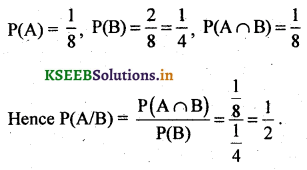Question 38.
The Probability that a MBA aspirant will join IIM is $$\frac{2}{5}$$ and that he will join XLRI is $$\frac{1}{3}$$. Find the Probability that (a) he will join IIM or XLRI (b) he will join neither IIM or XLRI.
Let A = He will join IIM
B = He will join XLRI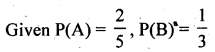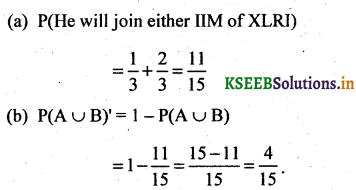Question 39.
One card is drawn from a pack of 52 cards. Find the Probability that
(a) Card is neither an ace nor a King
(b) Queen given the card is red
(c) Card is either black or a jack
(d) it is a face card.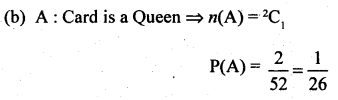Question 40.
A couple appears in an interview for two vacancies in the same post. The Probability of husbands selection is $$\frac{1}{7}$$ and the probability of wife’s selection is $$\frac{1}{8}$$. What is the Probability that (i) both of them are selected (ii) only one of them will be selected (iii) none of them will be selected (iv) only wife is selected.(ii) P (Only one of them will be selected = P(A). P(B’) + P(B). P(A’)
[Latex]=\frac{1}{7} \times \frac{4}{5}+\frac{1}{5} \times \frac{6}{7}=\frac{4}{35}+\frac{6}{35}=\frac{10}{35}=\frac{2}{7}$$(iii) P(none of them will be selected) = P(A’) x P(B’) [latex]\frac{6}{7} \times \frac{4}{5}=\frac{24}{35}$$

(iv) P(Wife only is selected) = P(B) x P(A’)
$$\frac{1}{5} \times \frac{6}{7}=\frac{6}{35}$$

Question 41.
From 8 gentlemen and 7 Ladies a committee of 5 is to be formed. What is the Probability that this committee consists of (i) Exactly 2 Ladies (ii) Atleast 3 gentlemens.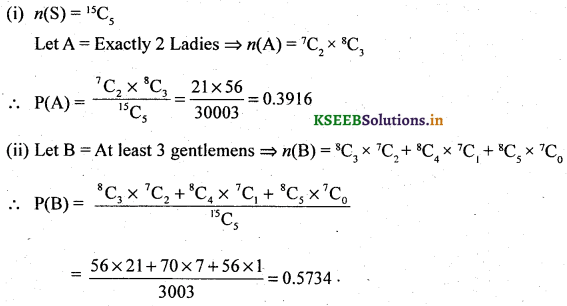Question 42.
A bag contains 6 red, 4 white and 2 black balls 2 balls are drawn at random. What is the probability that the balls are (i) both red (ii) 1 white and 1 black (iii) same colour (iv) different colour.
(i) n(S) = (6 + 4 + 2)C2 = 12C2
Let A = both red ⇒ n(A) = 6C2Question 43.
A die is rolled. If the outcome is an odd number. What is the probability that it is a 1 number greater than 1.
∴ $$P(A)=\frac{5}{6}$$